# Covariant tensor

(diff) ← Older revision | Latest revision (diff) | Newer revision → (diff)

of valency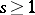A tensor of type, an element of the tensor productofcopies of the dual space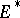of the vector spaceover a field. The spaceis itself a vector space overwith respect to the addition of covariant tensors of the same valency and multiplication of them by scalars. Letbe finite dimensional, let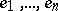be a basis ofand letbe the basis dual to it of. Then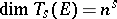and the set of all tensors of the form,, forms a basis for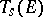. Any covariant tensor can be represented in the form. The numbersare called the coordinates, or components, of the covariant tensor relative to the basisof. Under a change of a basis ofaccording to the formulas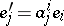and the corresponding change of the basis of, the components of the covariant tensorare changed according to the so-called covariant law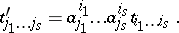If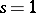, the covariant tensor is called a covariant vector; whena covariant tensor corresponds in an invariant way with an-linear mapping from the direct product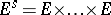(times) intoby taking the components of the covariant tensorrelative to the basisas the values of the-linear mappingat the basis vectors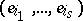in, and conversely; for this reason a covariant tensor is sometimes defined as a multilinear functional on.

For references see Covariant vector.

How to Cite This Entry:
Covariant tensor. Encyclopedia of Mathematics. URL: http://encyclopediaofmath.org/index.php?title=Covariant_tensor&oldid=13043
This article was adapted from an original article by I.Kh. Sabitov (originator), which appeared in Encyclopedia of Mathematics - ISBN 1402006098. See original article